# 自学Python5.5-面向对象三大基本特征_继承

### 1.1  继承概念

• 通过继承创建的新类称为“子类”或“派生类”。
• 被继承的类称为“基类”、“父类”或“超类”。
• 继承的过程，就是从一般到特殊的过程。要实现继承，可以通过“继承”（Inheritance）和“组合”（Composition）来实现。
• 一个子类可以被多个类继承。
• 一个子类可以继承多个父类（只有在python里面才有）。
class A(object):pass   # 父类，基类，超类
class B:pass   # 父类，基类，超类
class A_son(A,B):pass # 子类，派生类
class AB_son(A):pass # 子类，派生类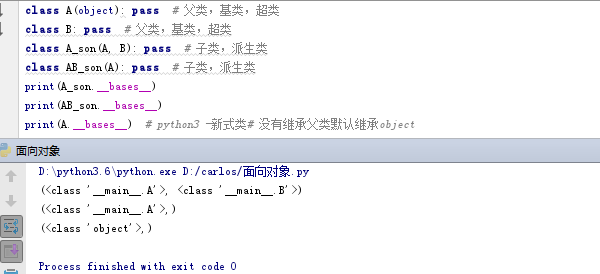### 1.2  继承与抽象（先抽象后继承）

# 比如举例人狗大战。 以下有两个类，你会发现两个类有共同点
class Dog:
def __init__(self,name,aggr,hp):
self.name = name
self.aggr = aggr
self.hp = hp
def bitc(self,person):
person.hp -= self.aggr
class person:
def __init__(self,name,aggr,hp,sex):
self.name = name
self.aggr = aggr
self.hp = hp
self.sex = sex
self.money = 0
def attack(self,dog):
person.hp -= self.aggr
def get_weapon(self,weapon):
if self.money >= weapon.price:
self.money -= weapon.price
self.get_weapon = weapon
self.aggr += weapon.aggr
else:
print("余额不足，请先充值")　　

将以上两个类相同的地方创建一个新的类， 两个类在继承新的类。

class Animal:
def __init__(self,name,aggr,hp):
self.name = name  #人和狗都有自己的昵称
self.aggr = aggr  #人和狗都有自己的攻击力
self.hp = hp      #人和狗都有自己的生命值
class Dog(Animal):
def bitc(self,person):
person.hp -= self.aggr
class person(Animal):
pass
Liu = Dog("刘老板",200,500)
print(Liu.name)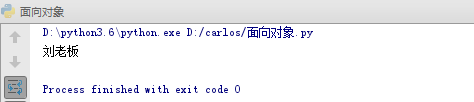class Animal:
def __init__(self):
print('执行Animal.__init__')
self.func()
def eat(self):
print('%s eating'%self.name)
def drink(self):
print('%s drinking'%self.name)
def func(self):
print('Animal.func')
class Dog(Animal):
def guard(self):
print('guarding')
def func(self):
print('Dog.func')
class Bird(Animal):
def __init__(self,name):
self.name = name
def lay(self):
print('laying')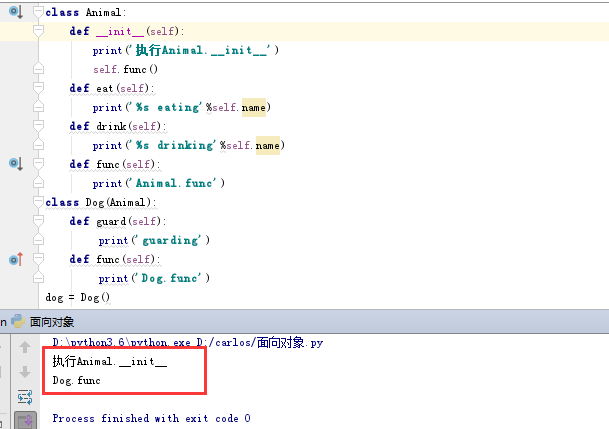### 1.3  派生

# 比如接上面的举例。
class Animal:
def __init__(self,name,aggr,hp):
self.name = name
self.aggr = aggr
self.hp = hp
class Dog(Animal):
def __init__(self,name,aggr,hp,kind):
self.kind = kind
def bitc(self,person):
person.hp -= self.aggr
Liu = Dog("刘老板",20,500,"吉娃娃")
print(Liu.name)     # 报错，因为只执行Dog类里面的_init_,但是里面没有name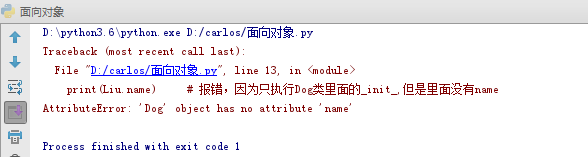class Animal:
def __init__(self,name,aggr,hp):
self.name = name
self.aggr = aggr
self.hp = hp
def eat(self):
print('吃药回血')
self.hp+=100
class Dog(Animal):
def __init__(self,name,aggr,hp,kind):
Animal.__init__(self,name,aggr,hp)  #
self.kind = kind       # 派生属性（父类没有的，子类新增的属性）
class Person(Animal):
def __init__(self,name,aggr,hp,sex):
Animal.__init__(self,name,aggr,hp)
self.sex = sex       # 派生属性
self.money = 0       # 派生属性
Liu = Dog('刘老板',20,500,'吉娃娃')
Liu.eat()       #使用的继承父类animal的eat方法
print(Liu.hp)
tong = Person('tong',1,2,None)
tong.eat()      #使用的继承父类animal的eat方法
print(tong.hp)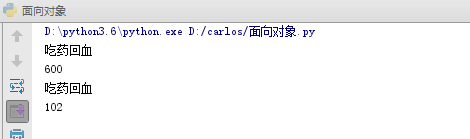class Animal:
def __init__(self,name,aggr,hp):
self.name = name
self.aggr = aggr
self.hp = hp
def eat(self):
print('吃药回血')
self.hp+=100
class Dog(Animal):
def __init__(self,name,aggr,hp,kind):
Animal.__init__(self,name,aggr,hp)  #
self.kind = kind       # 派生属性（父类没有的，子类有的属性）
def bite(self,person):     # 派生方法（父类没有的，子类有的方法）
person.hp -= self.aggr
class Person(Animal):
def __init__(self,name,aggr,hp,sex):
Animal.__init__(self,name,aggr,hp)
self.sex = sex       # 派生属性
self.money = 0       # 派生属性
def attack(self,dog):
dog.hp -= self.aggr
def get_weapon(self,weapon):
if self.money >= weapon.price:
self.money -= weapon.price
self.weapon = weapon
self.aggr += weapon.aggr
else:
print("余额不足，请先充值")
Liu = Dog('刘老板',20,500,'吉娃娃')
Liu.eat()       #使用的继承父类animal的eat方法
print(Liu.hp)

tong = Person('tong',1,2,None)
tong.eat()      #使用的继承父类animal的eat方法
print(tong.hp)

Liu.bite(tong) #使用Dog类自己的派生方法
print(tong.hp)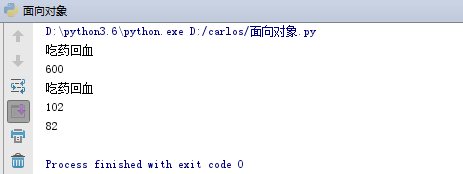class A:
def hahaha(self):
print('A')

class B(A):
def hahaha(self):
super().hahaha()
#super(B,self).hahaha()
#A.hahaha(self)
print('B')

a = A()
b = B()
b.hahaha()
super(B,b).hahaha()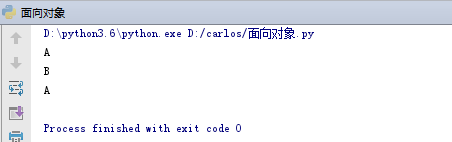总结：

• 父类中没有的属性 在子类中出现 叫做派生属性
• 父类中没有的方法 在子类中出现 叫做派生方法
• 只要是子类的对象调用，子类中有的名字 一定用子类的，子类中没有才找父类的，如果父类也没有报错
• 如果父类、子类都有，使用子类的
•      如果还想用父类的，单独调用父类的
•      父类名.方法名，需要自己传self参数
•      super().方法名，不需要自己传只在新式类中有，python3中所有类都是新式类
• 正常的代码中，单继承 === 减少了代码的重复
• 继承表达的是一种 子类是父类的关系
class Animal:
def __init__(self,name,aggr,hp):
self.name = name
self.aggr = aggr
self.hp = hp
def eat(self):
print('吃药回血')
self.hp+=100
class Dog(Animal):
def __init__(self,name,aggr,hp,kind):
super().__init__(name,aggr,hp)    #等价于Animal.__init__(self,name,aggr,hp)，不需要传self
self.kind = kind       # 派生属性（父类没有的，子类新增的属性）
Liu = Dog('刘老板',20,500,'吉娃娃')
Liu.eat()          #使用的继承父类animal的eat方法
print(Liu.hp)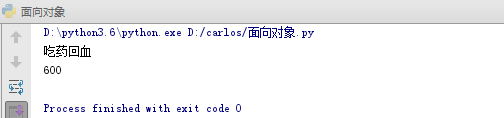super（）可以在类内使用，也可以在类外使用。

• super（）在类内使用：不需要传默认参数，就是类名和self
• super（）在类外使用：必须传类名和对象名
#super（）在类外和类内
class Animal:
def __init__(self,name,aggr,hp):
self.name = name
self.aggr = aggr
self.hp = hp
def eat(self):
print('吃药回血')
self.hp+=100
class Dog(Animal):
def __init__(self,name,aggr,hp,kind):
super().__init__(name,aggr,hp)    #等价于Animal.__init__(self,name,aggr,hp)
self.kind = kind       # 派生属性（父类没有的，子类新增的属性）
def eat(self):print("dog eating")
Liu = Dog('刘老板',20,500,'吉娃娃')
print(Liu.name)
Liu.eat()             # 执行的是Dog类里面的def eat(self):print("dog eating")
super(Dog,Liu).eat()  # 执行的是父类里面的def eat(self):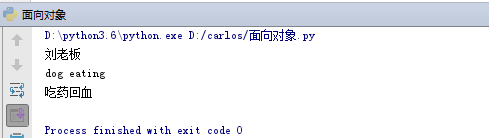## 二、多继承

class A:
def func(self):print("A")
class B:
def func(self):print("B")
class C:
def func(self):print("C")
class D(A,B,C):  # D类继承A,B,C，多继承
def func(self):print("D")

d = D()
d.func()   #此时调用的D类的func()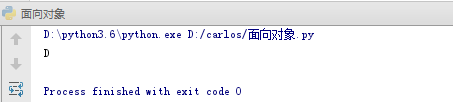class A:
def func(self):print("A")
class B:
def func(self):print("B")
class C:
def func(self):print("C")
class D(A,B,C):  # D类继承A,B,C，多继承
pass
#def func(self):print("D")

d = D()
d.func()   #此时调用的A类的func(),因为class D(A,B,C),A类离D最近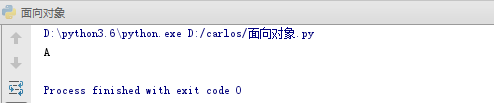### 2.1 多继承---经典案例----菱形继承（钻石继承）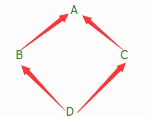B、C继承A，D继承B、C

class A:
def func(self):print("A")
class B(A):   # B类继承A
def func(self):print("B")
class C(A):   # C类继承A
def func(self):print("C")
class D(B,C):  # D类继承B,C
pass
#def func(self):print("D")

d = D()
d.func()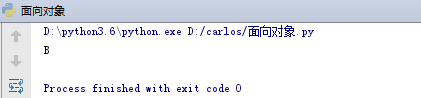class A:
def func(self):print("A")
class B(A):   # B类继承A
pass
#def func(self):print("B")
class C(A):   # C类继承A
def func(self):print("C")
class D(B,C):  # D类继承B,C
pass
#def func(self):print("D")

d = D()
d.func()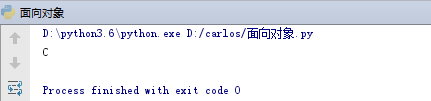class A:
def func(self):print("A")
class B(A):   # B类继承A
pass
#def func(self):print("B")
class C(A):   # C类继承A
pass
#def func(self):print("C")
class D(B,C):  # D类继承B,C
pass
#def func(self):print("D")

d = D()
d.func()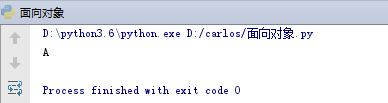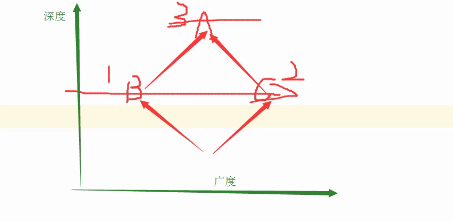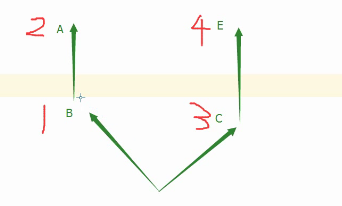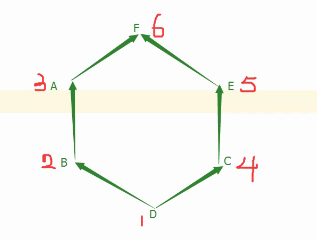### 2.2  总结

• 经典类中 深度优先
• 新式类中 广度优先

① python2.7 新式类和经典类共存，新式类要继承object

② python3 只有新式类，默认继承object

③ 经典类和新式类还有一个区别：mro方法只在新式类中存在，类名.mro方法，查看广度优先的继承顺序。

④ super 只在python3中存在

⑤ super的本质 ：不是单纯找父类 而是根据调用者的节点位置的广度优先顺序来的

class A(object):
def func(self): print('A')

class B(A):
def func(self):
super().func()
print('B')

class C(A):
def func(self):
super().func()
print('C')

class D(B,C):
def func(self):
super().func()
print('D')

b = D()
b.func()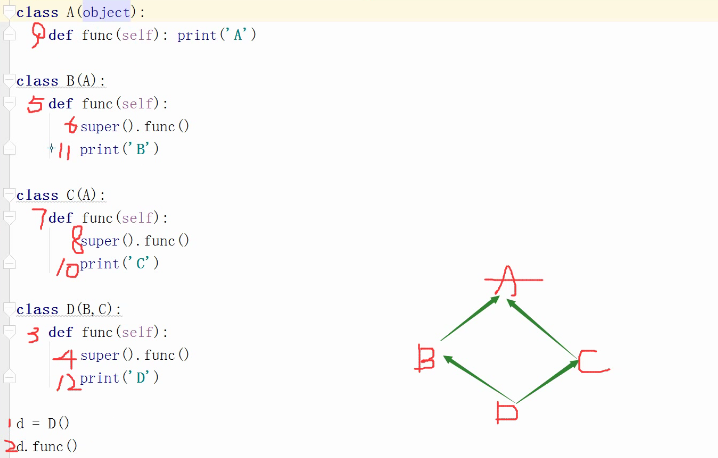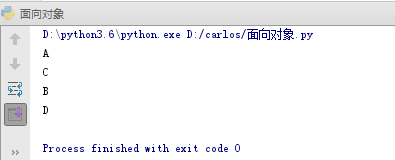......

posted on 2019-08-12 17:25  CARLOS_CHIANG  阅读(...)  评论(...编辑  收藏